# The { Odd-On-Odd Format } for { 'odd numbers ' }

#### Top Of Page

 Section I Introduction & Key Findings Section II The { Odd-On-Odd Format } Section III Studying { Divisibility by [ 3 ] } Section IV Concluding Remarks

## Introduction :

While working on the { 120-degrees Triangle } , this idea on the { Odd-On-Odd Format } came up , and here it is .

## Key Findings :

• ### For any { positive 'odd' integer } :

the associated { binary code } of [ 1's ] & [ 0's ] can be readily converted to :

• an { Odd-On-Odd Format } of [ red-color-balls ] & [ blue-color-balls ] ,

so that :

• the value of the { positive integer } may be evaluated via an expansion of the { Odd-On-Odd Equation } .

This then pave-the-way for an alternate interpretation to any { binary code } .

### A quick example here , for the number [ 93 ] :

• take the { binary format } for [ 93 ] , at 7-digits :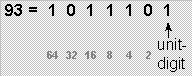• take the { 6-digits } to-the-left of the [ unit-digit ] to form the { red-color / blue-color code } , as follows :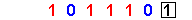• covert these into a { red-color-ball / blue-color-ball code } , and this is the { Odd-On-Odd Format } :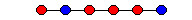• bring-in the general { Odd-On-Odd Equation } for a [ 6 color-balls code ] , as follows :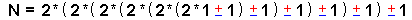• apply the [ 6 color-balls code ] to it to arrive at :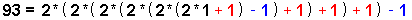• ( a [ +1 ] for a { red-color ball } & a [ -1 ] for a { blue-color ball } . )

The key features here are :

• the expression inside of any pair of brackets always evaluates to a { positive 'odd' integer } ,

• and hence the name { Odd-On-Odd } .

• the number [ 3 ] is always involved and [ 3 ] is therefore the 'root' of all expansions .

We have , therefore , found an alternate method for expanding the { 7-digits binary code } .

• ### Divisibility by [ 3 ] :

We also investigated { Divisibility by [ 3 ] } using :

• the { Base-10 Format } ,
• the { Binary Format } , &
• the { Odd-On-Odd Format } .

While we did move from-left-to-right on a { Binary Code } for the { Odd-On-Odd Format } ,

• our findings here tend to indicate that a tremendous savings may be achieved here ,

when we try to determine if a { number } is divisible by a particular { prime number } .

This can be an important issue in { factorization } !